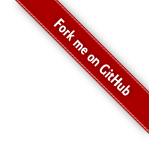# 中山纪念中学 培训 日记

ps奶一口：本宝宝觉得是不是周末的饭菜没有工作日的好吃呢？

“学生好，一切都好。”(我们学校的校训)

正经重要的  我想听的知识点讲的真的不错呢

“所以，小可爱要加油啊，抱抱~”

“**的夕阳也很美，只有你们**看不见太阳好吧”

$$\text{是的呢。 夕阳真的很美}$$

$$\text{只是， 我从来都没有见过呢}$$

$$\text{可能}$$

$$\text{我……我也没有机会}$$

$$\text{再看到了吧}$$

$$\text{除非，}$$

$$\text{除非明年七月份我还能来到这里。}$$

$$“\text{希望再明年NOI的赛场上看到你的身影}”$$

$$\text{是啊，我也希望。}$$

$$\text{我觉得，我可能随时会从这里跳下去吧。}$$

$$\text{但是，我希望}$$

$$\text{希望你不要拦着我}$$

$$\text{“好”}$$

posted @ 2018-08-09 22:37  米罗偕涯  阅读(1091)  评论(8编辑  收藏  举报